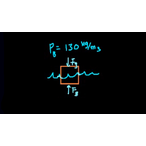# Class 11 Physics (India): Buoyant force example problems

Need more information? Get more details on the site of the provider.### Description

A couple of problems involving Archimedes' principle and buoyant forces.

## Class 11 Physics (India)

Let's learn, practice, and master topics of class 11 physics (NCERT) starting with kinematics and then moving to dynamics with Newton's laws of motion, work, energy, and power. Let's then use these as the foundation to learn about centre of mass, rotational motion, gravitation, solids, fluids, thermodynamics, and oscillations and waves.

## Buoyant force and Archimedes' principle

In these videos and articles you'll learn how pressure variations cause a buoyant force on a submerged object and how to calculate the value of the buoyant force. You'll also learn the meaning of Archimedes' principle.

## Topi…

There are no frequently asked questions yet. Send an Email to info@springest.com

A couple of problems involving Archimedes' principle and buoyant forces.

## Class 11 Physics (India)

Let's learn, practice, and master topics of class 11 physics (NCERT) starting with kinematics and then moving to dynamics with Newton's laws of motion, work, energy, and power. Let's then use these as the foundation to learn about centre of mass, rotational motion, gravitation, solids, fluids, thermodynamics, and oscillations and waves.

## Buoyant force and Archimedes' principle

In these videos and articles you'll learn how pressure variations cause a buoyant force on a submerged object and how to calculate the value of the buoyant force. You'll also learn the meaning of Archimedes' principle.

## Topic: Science

Learn about all the sciences, from physics, chemistry and biology, to cosmology and astronomy, across hundreds of videos, articles and practice questions.

There are no reviews yet.